JEE  >  WBJEE Physics Test - 4

# WBJEE Physics Test - 4

Test Description

## 40 Questions MCQ Test WBJEE Sample Papers, Section Wise & Full Mock Tests | WBJEE Physics Test - 4

WBJEE Physics Test - 4 for JEE 2022 is part of WBJEE Sample Papers, Section Wise & Full Mock Tests preparation. The WBJEE Physics Test - 4 questions and answers have been prepared according to the JEE exam syllabus.The WBJEE Physics Test - 4 MCQs are made for JEE 2022 Exam. Find important definitions, questions, notes, meanings, examples, exercises, MCQs and online tests for WBJEE Physics Test - 4 below.
Solutions of WBJEE Physics Test - 4 questions in English are available as part of our WBJEE Sample Papers, Section Wise & Full Mock Tests for JEE & WBJEE Physics Test - 4 solutions in Hindi for WBJEE Sample Papers, Section Wise & Full Mock Tests course. Download more important topics, notes, lectures and mock test series for JEE Exam by signing up for free. Attempt WBJEE Physics Test - 4 | 40 questions in 60 minutes | Mock test for JEE preparation | Free important questions MCQ to study WBJEE Sample Papers, Section Wise & Full Mock Tests for JEE Exam | Download free PDF with solutions
 1 Crore+ students have signed up on EduRev. Have you?
WBJEE Physics Test - 4 - Question 1

### If λ0 is the de-Broglie wavelength for a proton accelerated through a potetial difference of 100 V, the de-Broglie wavelength for α -particle accelerated through the same potential difference is

WBJEE Physics Test - 4 - Question 2

### The number of dry cells, each of e.m.f. 1.5 volt and internal resistance 0.5 ohm that must be joined in series with a resistance of 20 ohm so as to send a current of 0.6 ampere through the circuit is

WBJEE Physics Test - 4 - Question 3

### The resistance of a coil is 4.2 Ω at 100ºC and the temperature coefficient of resistance of its material is 0.004/ºC. Its resistance at 0ºC is

WBJEE Physics Test - 4 - Question 4

A body of mass 0.05kg is observed to fall with an acceleration of 9.5ms-2. The opposing force of air on the body is........(g = 9.8ms-2)

WBJEE Physics Test - 4 - Question 5

The electric field inside a charged conductor of radius r is

WBJEE Physics Test - 4 - Question 6

The reason for the change in shape of a regular body is

WBJEE Physics Test - 4 - Question 7

Power delivered by the source in the circuit is maximum, when

WBJEE Physics Test - 4 - Question 8

Acceleration due to gravity is 'g' on the surface of the earth. The value of acceleration due to gravity at a height of 32 km above earth's surface is

WBJEE Physics Test - 4 - Question 9

Two identical conducting plates of plate area A are separated by a distance d to form a parallel plate air capacitor. Now a metal sheet of thickness d/2 is inserted between the plates of the capacitor. The ratio of capacitance before the insertion of plate and after the insertion of plate is

Detailed Solution for WBJEE Physics Test - 4 - Question 9

Now capacitance of capacitor before inserting metal plate is
C = (ε0A)/d
And after the insertion of any dielectric plate filling the capacitor partially is
C = (ε0A)/[(d-t)+t/K]
We know that K for a metal is infinite thus
C = (ε0A)/(d-t) Here t=d/2
thus C’=(2ε0A)/d=2C
C:C’=1:2
Hence (a) is the correct choice

WBJEE Physics Test - 4 - Question 10

A galvanometer has a resistance of 25 Ω and a maximum of 0.01 A current can be passed through it. In order to change it into an ammeter of range 10 A, the shunt resistance required is

WBJEE Physics Test - 4 - Question 11

To convert a galvanometer into a voltmeter, we must connect a

WBJEE Physics Test - 4 - Question 12

At the neutral point, horizontal component of the magnetic field due to a magnet is

WBJEE Physics Test - 4 - Question 13

Hydraulic press is based on

WBJEE Physics Test - 4 - Question 14

A car moves for half of its time at 80 km/h and for rest half of time at 40 km/h. Total distance covered is 60 km. What is the average speed of the car?

WBJEE Physics Test - 4 - Question 15

A stone is just released from the window of a train moving along a horizontal straight track. The stone will hit the ground following

WBJEE Physics Test - 4 - Question 16

A cricketer can throw a ball to a maximum horizontal distance of 100 m. The speed with which he throws the ball is (to the nearest integer)

WBJEE Physics Test - 4 - Question 17

Among electron, proton, neutron and α - particle the maximum penetration capacity is for

WBJEE Physics Test - 4 - Question 18

A mass m is vertically suspended from a spring of negligible mass. The system oscillates with a frequency n. What will be the frequency of the system, if a mass 4m is suspended from the same spring ?

WBJEE Physics Test - 4 - Question 19

A spring has a certain mass suspended from it and its period for vertical oscillation is T. The spring is now cut into two halves and the same mass is suspended from one of the halves. The period of verticle oscillation is now

WBJEE Physics Test - 4 - Question 20

A circular disc is to be made by using iron aluminium, so that it acquires maximum moment of inertia about its geometrical axis. It can be obtained with

WBJEE Physics Test - 4 - Question 21

A ray of light is incident on a surface of glass slab at an angle 450 . If the lateral shift produced per unit thickness is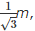the angle of refraction produced is

Detailed Solution for WBJEE Physics Test - 4 - Question 21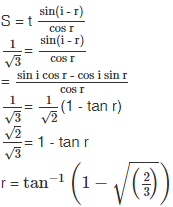WBJEE Physics Test - 4 - Question 22

A couple produces

WBJEE Physics Test - 4 - Question 23

The intrinsic semiconductor becomes an insulator at

WBJEE Physics Test - 4 - Question 24

Platinum and silicon are heated upto 250ºC and after then cooled. In the process of cooling

WBJEE Physics Test - 4 - Question 25

70 calories of heat are required to raise the temperature of 2 moles of an ideal gas at constant pressure from 30ºC to 35ºC. The amount of heat required to raise the temperature of the same sample of the gas through the same range at constant volume is nearly (Gas constant = 1.99 cal/K-mole)

WBJEE Physics Test - 4 - Question 26

In adiabatic expansion of a gas

WBJEE Physics Test - 4 - Question 27

Which of the following pair of physical quantities had same dimensional formula?

WBJEE Physics Test - 4 - Question 28

If a,b,c are any three vectors, the statement is true

WBJEE Physics Test - 4 - Question 29

Who was the first to demonstrate the phenomenon of interference of light?

WBJEE Physics Test - 4 - Question 30

The circuit shown in the figure contains two diodes each with a forward resistance of 50 ohms and with infinite backward resistance .If the battery voltage is 6V, the current through the 100 ohm resistance (in amperes) is

WBJEE Physics Test - 4 - Question 31

A body is moved along a straight line by a machine delivering constant power. The distance moved by the body in time t is propotional to

WBJEE Physics Test - 4 - Question 32

The pulleys and strings shown in the figure are smooth and of negligible mass, For the system to remain in equilibrium the angle θ should be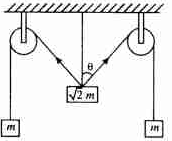WBJEE Physics Test - 4 - Question 33

The north pole of a magnet is brought near a metallic ring  .The direction of induced current in the ring will be

Detailed Solution for WBJEE Physics Test - 4 - Question 33

Correct option is

a

Anticlockwise

When we bring a north pole near a metallic ring applying Lenz's law the ring will behave as north pole so that repulsion occurs. So direction ofcurrent induced in it will be anticlockwiseas depicted above.WBJEE Physics Test - 4 - Question 34

A point source of light of power P and wavelength λ is emitting light in all direction. The number of photons present in a spherical region of radius r to radius 2r with centre at source, is

WBJEE Physics Test - 4 - Question 35

If the same instrument is to measure 25 V, the resistance to be connected in series is

WBJEE Physics Test - 4 - Question 36

A conducting square loop of the side L and resistance R moves in its plane with a uniform velocity v perpendicular to one of its sides. A magnetic induction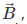constant in time and space, pointing perpendicular and into the plane of the loop exists everywhere. The current induced in the loop is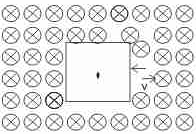WBJEE Physics Test - 4 - Question 37

A shell is fired from a cannon with a velocity v (m/sec.) at an angle θ with the horizontal direction. At the highest point in its path it explodes into two pieces of equal mass. One of the pieces retraces its path to the cannon and the speed (in m/sec) of the other piece immediately after the explosion is

*Multiple options can be correct
WBJEE Physics Test - 4 - Question 38

What are electromagnetic waves associated with photons?

*Multiple options can be correct
WBJEE Physics Test - 4 - Question 39

A satellite S is moving in an elliptical orbit around the earth. The mass of the satellite is very small compared to the mass of the earth.

WBJEE Physics Test - 4 - Question 40

Two very long, straight, parallel wires carry steady currents I and -I respectively. The distance between the wires is d. At a certain instant of time, a point charge q is at a point equidistant from the two wires, in the plane of the wires. Its instantaneous velocity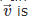is perpendicular to this plane. The magnitude of the force due to the magnetic field acting on the charge at this instant is

## WBJEE Sample Papers, Section Wise & Full Mock Tests

4 videos|10 docs|54 tests
 Use Code STAYHOME200 and get INR 200 additional OFF Use Coupon Code
Information about WBJEE Physics Test - 4 Page
In this test you can find the Exam questions for WBJEE Physics Test - 4 solved & explained in the simplest way possible. Besides giving Questions and answers for WBJEE Physics Test - 4, EduRev gives you an ample number of Online tests for practice

## WBJEE Sample Papers, Section Wise & Full Mock Tests

4 videos|10 docs|54 tests